Geometry 10-8 Geometric Probability
starstarstarstarstarstarstarstarstarstar
by Matthew Richardson
| 24 Questions
Note from the author:
A complete formative lesson with embedded slideshow, mini lecture screencasts, checks for understanding, practice items, mixed review, and reflection. I create these assignments to supplement each lesson of Pearson's Common Core Edition Algebra 1, Algebra 2, and Geometry courses. See also mathquest.net and twitter.com/mathquestEDU.
The outlined content above was added from outside of Formative.
The outlined content above was added from outside of Formative.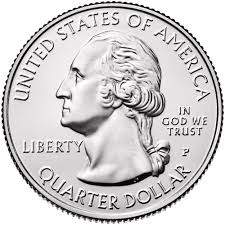1
10 pts
Sovle It! A fair coin is equally likely to land heads up or tails up. Suppose you toss a fair coin three times. What is the probability that the coin will land tails up exactly twice?

Enter your response as a reduced fraction in this form: "2/5"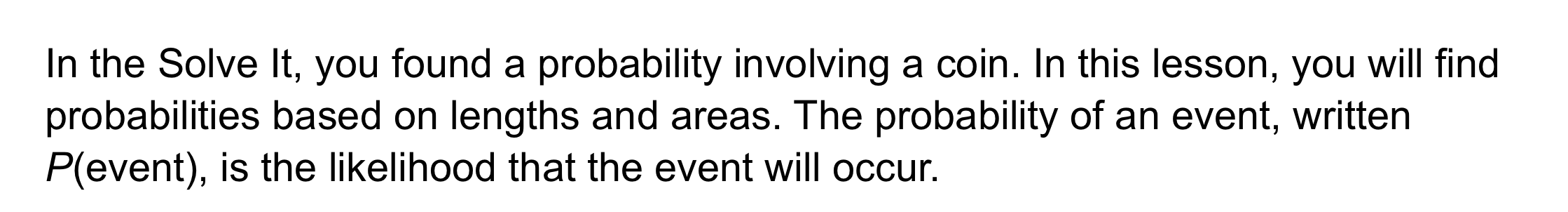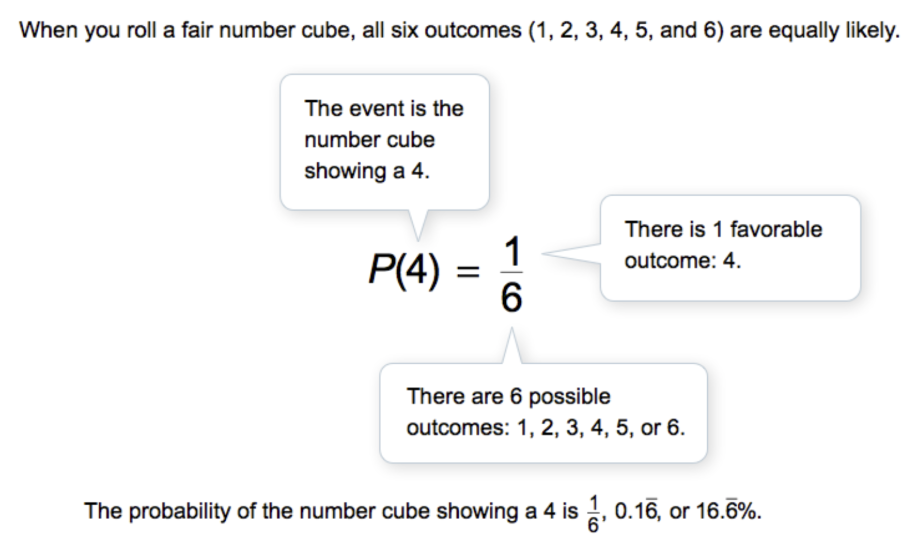The outlined content above was added from outside of Formative.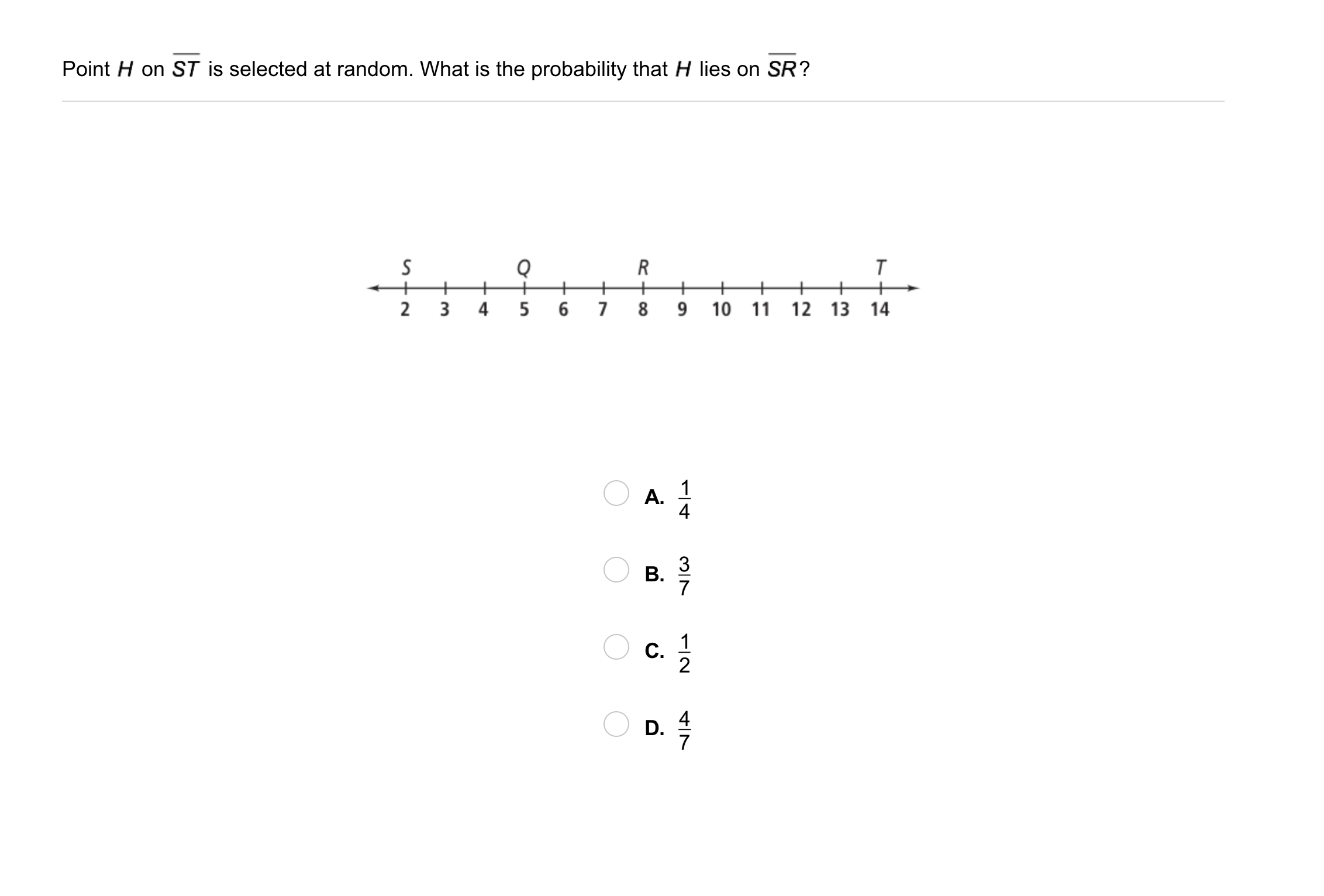2
2
10 pts
Problem 1 Got It?
A
B
C
D
The outlined content above was added from outside of Formative.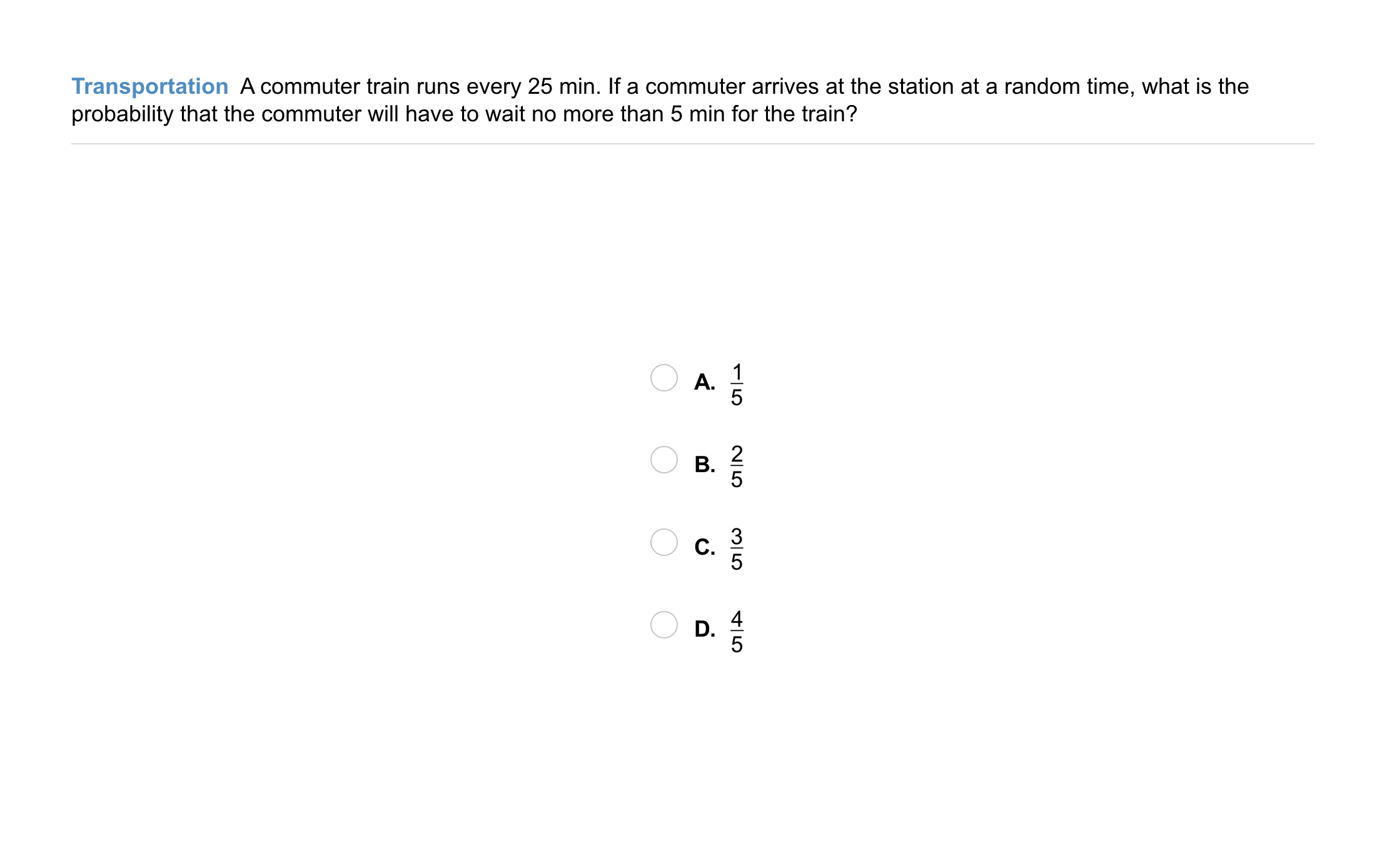3
3
10 pts
Problem 2 Got It?
A
B
C
DThe outlined content above was added from outside of Formative.4
4
10 pts
Problem 3 Got It?
A
B
C
D
The outlined content above was added from outside of Formative.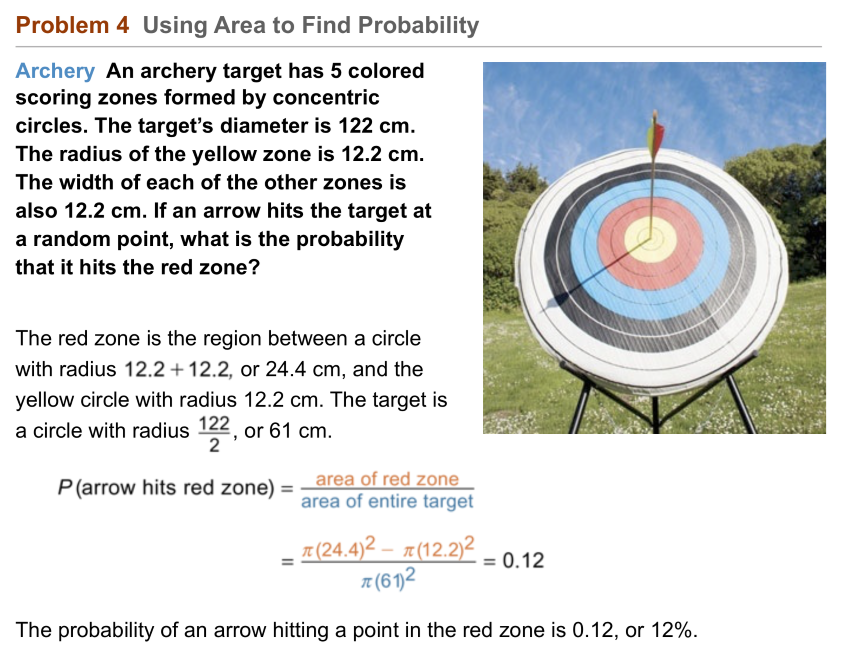5
6
5
10 pts
Problem 4 Got It? What is the probability that an arrow hits the yellow zone of the same target from Problem 4? Round your answer to the nearest percent and respond using this format: "12%"7
7
10 pts
A
B
C
D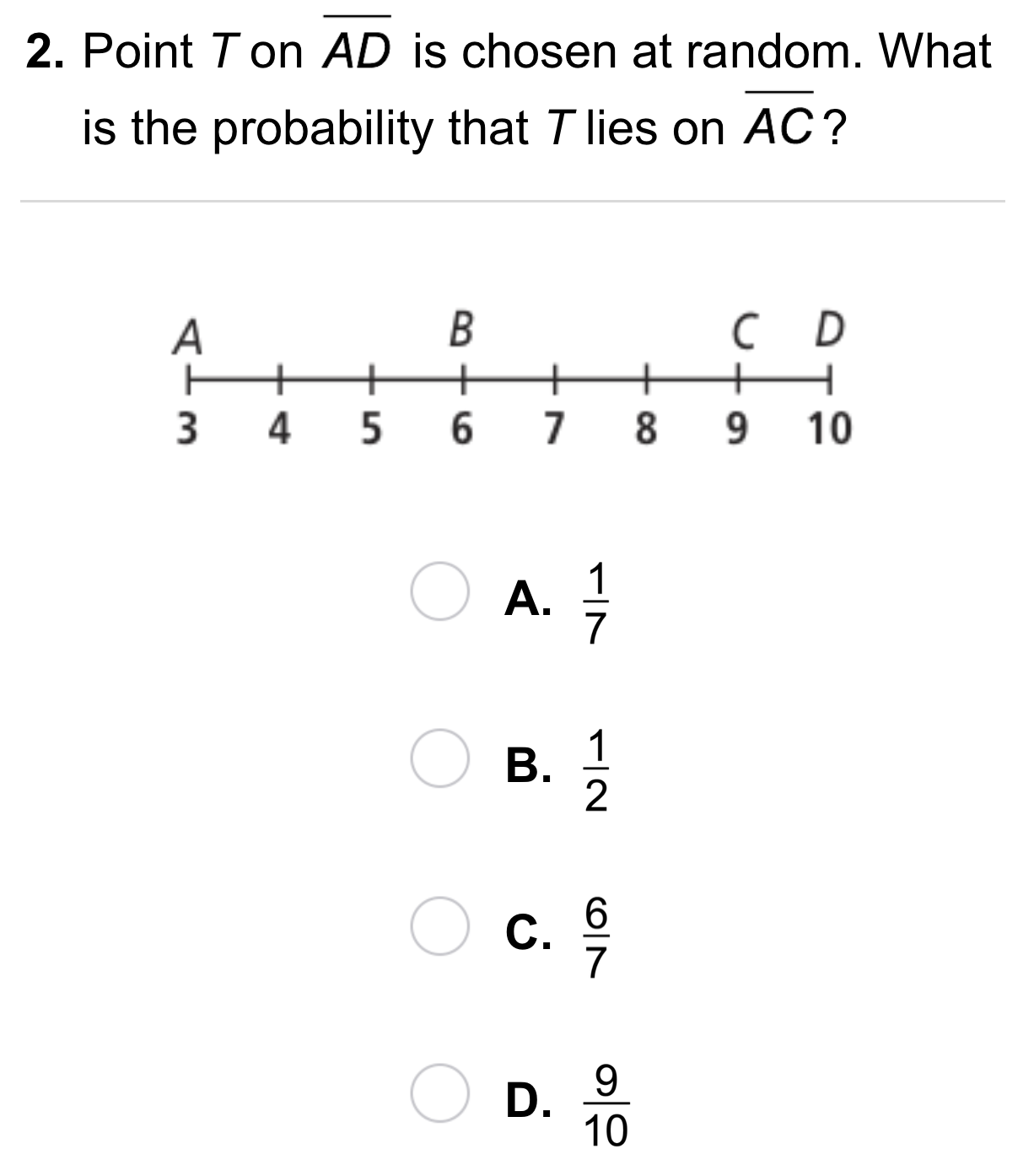8
8
10 pts
A
B
C
D9
9
10 pts
A
B
C
D10
10
10 pts
A
B
C
D11
11
10 pts
A
B
C
D12
12
10 pts
Reasoning: In the figure shown, SQ/QT = ½. What is the probability that a point on segment ST chosen at random will lie on segment QT? Enter your response as a reduced fraction in this form: "5/7"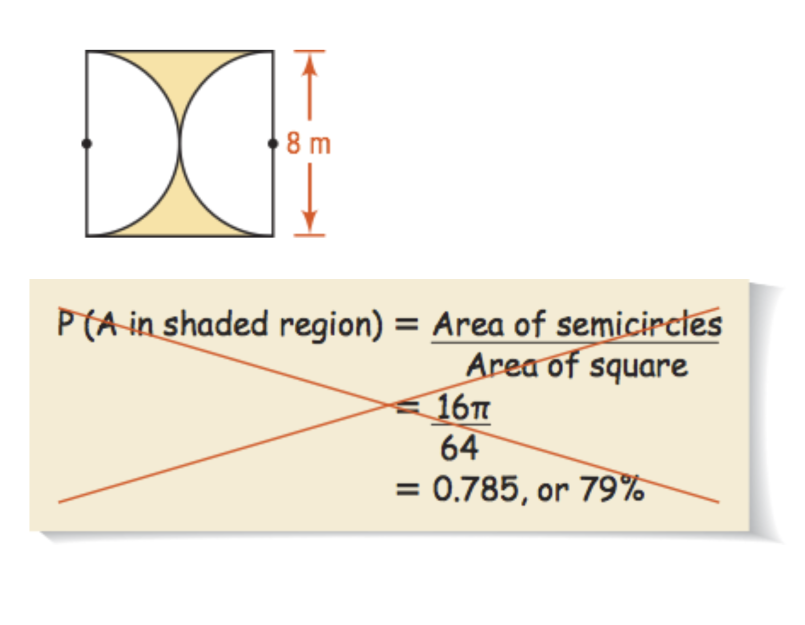13
13
10 pts
Error Analysis: Your class needs to find the probability that a point A in the square chosen at random lies in the shaded region. You classmate's work is shown. What is the error? Explain.14
10 pts
Review Lesson 10-7: A circle has circumference 20 π ft. What is the area in terms of π? Enter your response in this format: "43 pi"
15
10 pts
Review Lesson 10-7: A circle has radius 12 cm. What is the area of a sector of the circle with a 30° central angle in terms of π?
144π cm²
12π cm²
6π cm²
3π cm²16
10 pts
Review Lesson 9-4: Use △ABC above to match the coordinates of the image, △A''B''C'', that results from each composition of transformations described.

• A'' = (-6, -1), B'' = (-5, -4), C'' = (-3, -2)
• A'' = (-2, -2), B'' = (1, -1), C'' = (-1, 1)
• A'' = (4, -1), B'' = (1, 0), C'' = (3, 2)
17
10 pts
Review Lesson 1-2: Use the cube provided on the canvas and shade the planes containing the indicated points using the indicated colors.
18
10 pts
Review Lesson 1-2: Draw a net for the cube in the space provided. Label all vertices.19
5 pts
Vocabulary Review: A point indicates a location and has no size.
True
False
20
5 pts
Vocabulary Review: A line contains a finite number of points.
True
False21
21
5 pts
Vocabulary Review: Which segment in the diagram includes point S?22
5 pts
Use Your Vocabulary: Select the correct order of the terms favorable and possible to fill in the blanks and complete the sentence.

The probability of an event is the ratio of the number of __?__ outcomes to the number of __?__ outcomes.
favorable, possible
possible, favorable
23
10 pts
Use Your Vocabulary: There are 7 red marbles and 3 green marbles in a bag. One marble is chosen at random. Identify the probability that a green marble is chosen, written as a fraction, as a decimal, and as a percent. Each category will contain only one probability.
• 3/7
• 3/10
• 0.03
• 0.30
• 30%
• 70%
• Written as a fraction
• Written as a decimal
• Written as a percent
24
10 pts
Reflection: Math Success